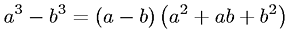Equations > Algebra > Polynomials > Difference of Cubes Factorization

### Difference of Cubes FactorizationLatex Code:

MathML Code:

 ${a}^{3}-{b}^{3}=\left(a-b\right)\left({a}^{2}+\mathrm{ab}+{b}^{2}\right)$

MathType 5.0: